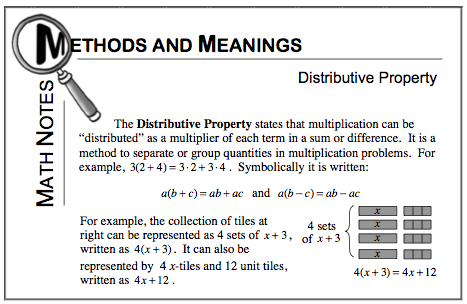### Home > MC2 > Chapter 5 > Lesson 5.2.1 > Problem5-46

5-46.
1. Copy and rewrite the following expressions by combining like terms, making zeros, and using the Distributive Property. Visualizing the expressions by using algebra tiles may be helpful. Homework Help ✎

1. (–3) + 4x + 2 + 2x + 2x

2. –8x + 4 + (–3)

3. 7x2 + 3x + 4 + 7x2 + 3x + 4

4. 5(x – 4)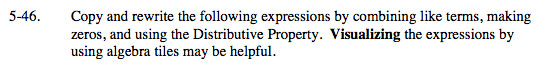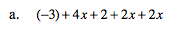Group like terms together.

( 4x + 2x + 2x ) + ( −3 + 2 )
Now simplify.

8x − 1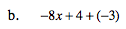Visualize eight negative x algebra tiles. What happens when you add 4 unit tiles but then take 3 of these unit tiles away?

−8x + 1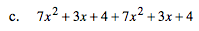See part (a).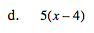Refer to the Math Notes box of Lesson 5.1.3.